# DAV Class 6 Maths Chapter 3 Worksheet 2 Solutions

The DAV Class 6 Maths Book Solutions Pdf and DAV Class 6 Maths Chapter 3 Worksheet 2 Solutions of Integers offer comprehensive answers to textbook questions.

## DAV Class 6 Maths Ch 3 WS 2 Solutions

Question 1.
(a) Mark any point as origin on the given number line.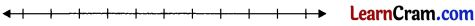Solution: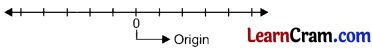(b) Write integers on either side of the origin with proper signs.
Solution: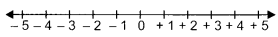Question 2.
(a) 3, – 1
Solution: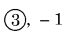(b) 0, – 8
Solution:(c) – 6, – 4
Solution: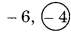(d) 14, – 7
Solution:(e) – 9, – 8
Solution: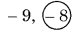(f) 4, 7
Solution: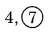Question 3.
Write all the integers between:
(a) – 5 and 0
Solution:
Integers between – 5 and 0 are – 4, – 3, – 2, – 1

(b) – 4 and 3
Solution:
Integers between – 4 and 3 are 3, – 2, – 1, 0, 1, 2

(c) – 11 and 1
Solution:
Integers between – 11 and 1 are – 10,- 9,- 8, – 7, – 6,- 5, – 4, – 3, – 2, – 1, 0

(d) – 6 and – 1
Solution:
Integers between – 6 and – 1 are – 5, – 4, – 3, – 2Question 4.
Compare the numbers and insert an appropriate symbol (<, >, =) in the given space.

(a) 3 ____ – 3
Solution:
3 > -3

(b) – 1 ____ 0
Solution:
– 1 < 0

(c) – 101 ____ – 104
Solution:
– 101 > – 104

(d) – 82 ____ – 28
Solution:
– 82 > – 88

(e) – 4 ____ – 14
Solution:
– 4 > – 14

(f) 16 ____ – 16
Solution:
16 > – 16

(g) – 97 ____ – 98
Solution:
– 97 > – 98

(h) – 197 ____ – 96
Solution:
– 197 < – 96

(i) 0 ____ – 7
Solution:
0 > – 7

(j) – 1 ____ 1
Solution:
– 1 < 1Question 5.Solution: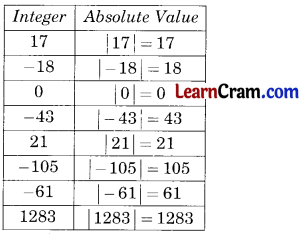Question 6.
Write the following in ascending order.

(a) 4, – 5, 16, – 11, – 21, 50
Solution:
Ascending order is – 21 < – 11 < – 5 < 4 < 16 < 50

(b) 0, – 1, 7, – 16, – 12, – 30
Solution:
Ascending order is – 30 < – 16 <-12<- 1<0<7

Question 7.
Write the following in descending order
(a) – 171, 26, – 43, 103, – 105, 77
Solution:
Descending order is 103 > 77 > 26 > – 43 > – 105 > – 171

(b) 9, – 8, 0, – 75, – 79, 93
Solution:
Descending order is 93 > 9 > 0 > – 8> – 75 > – 79.Question 8.
Write ‘True’ or ‘False’ for the following statements.

(а) Every integer is either positive or negative.
Solution:
False

(b) Zero is greater than every negative integer.
Solution:
True

(c) An integer to the left of another integer is always smaller.
Solution:
True

(d) We can find the smallest integer.
Solution:
False

(e) Absolute value of a given integer is always greater than the integer.
Solution:
False(f) All natural numbers are positive integers.
Solution:
True

(g) All whole numbers are integers.
Solution:
True

(h) Absolute value of 3 is – 3.
Solution:
False